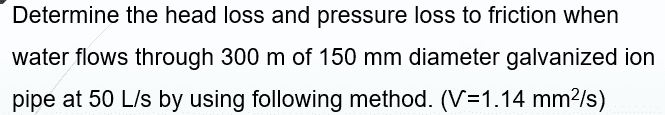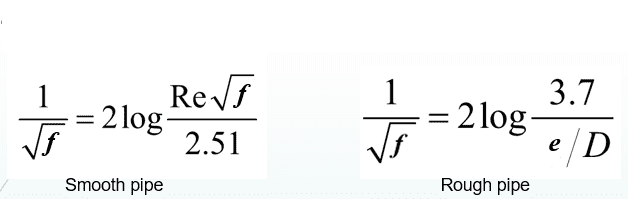# Von Karman and Prandtl laws

## Homework Statement

there are 2 formula of vont karman and prandit laws to find the friction factorfor rough pipe and smooth pipe , which formula should i use for this question?.
P/ s : I'm asked to find the friction factor using the vont karman and prandit laws.

## The Attempt at a Solution

it didnt stated whether it's smooth or rough pipe , which formula should we use?

BvU
Homework Helper
I was going to propose you do both (assuming some reasonable ##e## for galvanized ion pipe ) and compare ...On the other hand, you do have the data to calculate Re so I certainly wouldn't leave out that one...

•foo9008
I was going to propose you do both (assuming some reasonable ##e## for galvanized ion pipe ) and compare ...On the other hand, you do have the data to calculate Re so I certainly wouldn't leave out that one...
well , i have found that the f for rough pipe is 0.017 , nut i am not sure how to find f for smooth pipe using von karman and Prandlt laws ... for smooth pipe , i have 1 / ( surd f ) = 2 log [(3.72 x 10^5) (surd f ) / 2.51 ]

P/ s: by using scientific calculator , i get the f for smooth pipe is 0.014 , which value should i choose ? f= 0.017 or 0.014 ?

Nidum